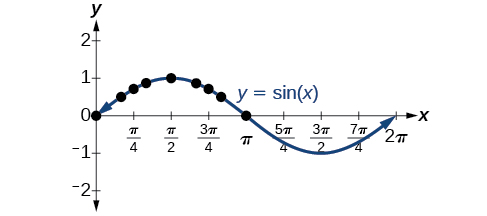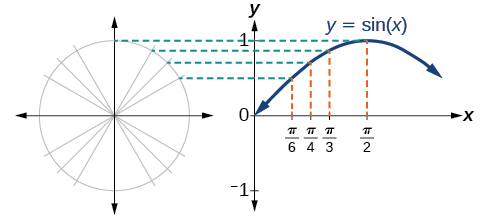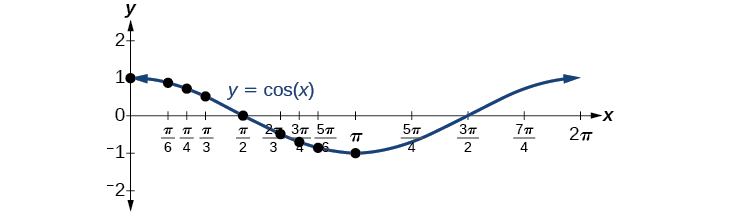# 8.1 Graphs of the sine and cosine functions

 Page 1 / 13
In this section, you will:
• Graph variations of  y=sin( x )  and  y=cos( x ).
• Use phase shifts of sine and cosine curves.Light can be separated into colors because of its wavelike properties. (credit: "wonderferret"/ Flickr)

White light, such as the light from the sun, is not actually white at all. Instead, it is a composition of all the colors of the rainbow in the form of waves. The individual colors can be seen only when white light passes through an optical prism that separates the waves according to their wavelengths to form a rainbow.

Light waves can be represented graphically by the sine function. In the chapter on Trigonometric Functions , we examined trigonometric functions such as the sine function. In this section, we will interpret and create graphs of sine and cosine functions.

## Graphing sine and cosine functions

Recall that the sine and cosine functions relate real number values to the x - and y -coordinates of a point on the unit circle. So what do they look like on a graph on a coordinate plane? Let’s start with the sine function    . We can create a table of values and use them to sketch a graph. [link] lists some of the values for the sine function on a unit circle.

 $x$ $0$ $\frac{\pi }{6}$ $\frac{\pi }{4}$ $\frac{\pi }{3}$ $\frac{\pi }{2}$ $\frac{2\pi }{3}$ $\frac{3\pi }{4}$ $\frac{5\pi }{6}$ $\pi$ $\mathrm{sin}\left(x\right)$ $0$ $\frac{1}{2}$ $\frac{\sqrt{2}}{2}$ $\frac{\sqrt{3}}{2}$ $1$ $\frac{\sqrt{3}}{2}$ $\frac{\sqrt{2}}{2}$ $\frac{1}{2}$ $0$

Plotting the points from the table and continuing along the x -axis gives the shape of the sine function. See [link] .The sine function

Notice how the sine values are positive between 0 and $\text{\hspace{0.17em}}\pi ,\text{\hspace{0.17em}}$ which correspond to the values of the sine function in quadrants I and II on the unit circle, and the sine values are negative between $\text{\hspace{0.17em}}\pi \text{\hspace{0.17em}}$ and $\text{\hspace{0.17em}}2\pi ,\text{\hspace{0.17em}}$ which correspond to the values of the sine function in quadrants III and IV on the unit circle. See [link] .Plotting values of the sine function

Now let’s take a similar look at the cosine function    . Again, we can create a table of values and use them to sketch a graph. [link] lists some of the values for the cosine function on a unit circle.

 $\mathbf{x}$ $0$ $\frac{\pi }{6}$ $\frac{\pi }{4}$ $\frac{\pi }{3}$ $\frac{\pi }{2}$ $\frac{2\pi }{3}$ $\frac{3\pi }{4}$ $\frac{5\pi }{6}$ $\pi$ $\mathbf{cos}\left(\mathbf{x}\right)$ $1$ $\frac{\sqrt{3}}{2}$ $\frac{\sqrt{2}}{2}$ $\frac{1}{2}$ $0$ $-\frac{1}{2}$ $-\frac{\sqrt{2}}{2}$ $-\frac{\sqrt{3}}{2}$ $-1$

As with the sine function, we can plots points to create a graph of the cosine function as in [link] .The cosine function

Because we can evaluate the sine and cosine of any real number, both of these functions are defined for all real numbers. By thinking of the sine and cosine values as coordinates of points on a unit circle, it becomes clear that the range of both functions must be the interval $\text{\hspace{0.17em}}\left[-1,1\right].$

In both graphs, the shape of the graph repeats after $\text{\hspace{0.17em}}2\pi ,\text{\hspace{0.17em}}$ which means the functions are periodic with a period of $\text{\hspace{0.17em}}2\pi .\text{\hspace{0.17em}}$ A periodic function    is a function for which a specific horizontal shift    , P , results in a function equal to the original function: $\text{\hspace{0.17em}}f\left(x+P\right)=f\left(x\right)\text{\hspace{0.17em}}$ for all values of $\text{\hspace{0.17em}}x\text{\hspace{0.17em}}$ in the domain of $\text{\hspace{0.17em}}f.\text{\hspace{0.17em}}$ When this occurs, we call the smallest such horizontal shift with $\text{\hspace{0.17em}}P>0\text{\hspace{0.17em}}$ the period    of the function. [link] shows several periods of the sine and cosine functions.

Looking again at the sine and cosine functions on a domain centered at the y -axis helps reveal symmetries. As we can see in [link] , the sine function    is symmetric about the origin. Recall from The Other Trigonometric Functions that we determined from the unit circle that the sine function is an odd function because $\text{\hspace{0.17em}}\mathrm{sin}\left(-x\right)=-\mathrm{sin}\text{\hspace{0.17em}}x.\text{\hspace{0.17em}}$ Now we can clearly see this property from the graph.

#### Questions & Answers

A laser rangefinder is locked on a comet approaching Earth. The distance g(x), in kilometers, of the comet after x days, for x in the interval 0 to 30 days, is given by g(x)=250,000csc(π30x). Graph g(x) on the interval [0, 35]. Evaluate g(5)  and interpret the information. What is the minimum distance between the comet and Earth? When does this occur? To which constant in the equation does this correspond? Find and discuss the meaning of any vertical asymptotes.
Kaitlyn Reply
The sequence is {1,-1,1-1.....} has
amit Reply
circular region of radious
Kainat Reply
how can we solve this problem
Joel Reply
Sin(A+B) = sinBcosA+cosBsinA
Eseka Reply
Prove it
Eseka
Please prove it
Eseka
hi
Joel
June needs 45 gallons of punch. 2 different coolers. Bigger cooler is 5 times as large as smaller cooler. How many gallons in each cooler?
Arleathia Reply
7.5 and 37.5
Nando
find the sum of 28th term of the AP 3+10+17+---------
Prince Reply
I think you should say "28 terms" instead of "28th term"
Vedant
the 28th term is 175
Nando
192
Kenneth
if sequence sn is a such that sn>0 for all n and lim sn=0than prove that lim (s1 s2............ sn) ke hole power n =n
SANDESH Reply
write down the polynomial function with root 1/3,2,-3 with solution
Gift Reply
if A and B are subspaces of V prove that (A+B)/B=A/(A-B)
Pream Reply
write down the value of each of the following in surd form a)cos(-65°) b)sin(-180°)c)tan(225°)d)tan(135°)
Oroke Reply
Prove that (sinA/1-cosA - 1-cosA/sinA) (cosA/1-sinA - 1-sinA/cosA) = 4
kiruba Reply
what is the answer to dividing negative index
Morosi Reply
In a triangle ABC prove that. (b+c)cosA+(c+a)cosB+(a+b)cisC=a+b+c.
Shivam Reply
give me the waec 2019 questions
Aaron Reply

### Read also:

#### Get the best Algebra and trigonometry course in your pocket!

Source:  OpenStax, Algebra and trigonometry. OpenStax CNX. Nov 14, 2016 Download for free at https://legacy.cnx.org/content/col11758/1.6
Google Play and the Google Play logo are trademarks of Google Inc.

Notification Switch

Would you like to follow the 'Algebra and trigonometry' conversation and receive update notifications?ByBy Lakeima RobertsBy Anonymous UserBy RhodesBy Abishek DevarajBy Edward Biton